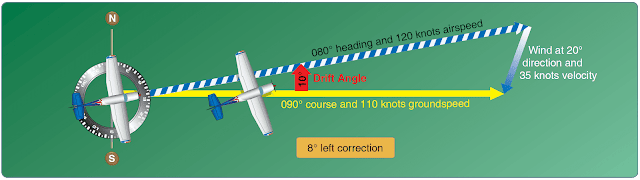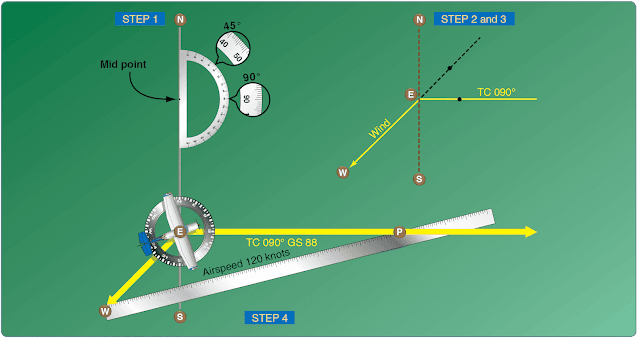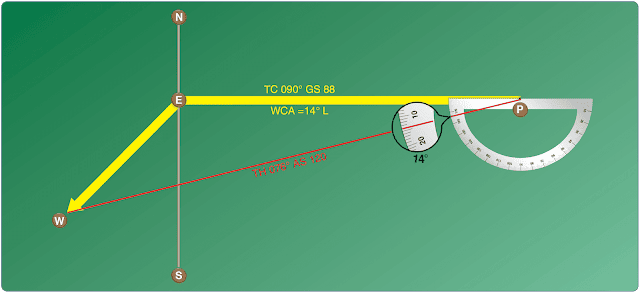Dead reckoning is navigation solely by means of computations based on time, airspeed, distance, and direction. The products derived from these variables, when adjusted by wind speed and velocity, are heading and GS. The predicted heading takes the aircraft along the intended path and the GS establishes the time to arrive at each checkpoint and the destination. Except for flights over water, dead reckoning is usually used with pilotage for cross-country flying. The heading and GS, as calculated, is constantly monitored and corrected by pilotage as observed from checkpoints.

## Wind Triangle or Vector Analysis

If there is no wind, the aircraft’s ground track is the same as the heading and the GS is the same as the true airspeed. This condition rarely exists. A wind triangle, the pilot’s version of vector analysis, is the basis of dead reckoning.

The wind triangle is a graphic explanation of the effect of wind upon flight. GS, heading, and time for any flight can be determined by using the wind triangle. It can be applied to the simplest kind of cross-country flight, as well as the most complicated instrument flight. The experienced pilot becomes so familiar with the fundamental principles that estimates can be made that are adequate for visual flight without actually drawing the diagrams. The beginning student, however, needs to develop skill in constructing these diagrams as an aid to the complete understanding of wind effect. Either consciously or unconsciously, every good pilot thinks of the flight in terms of wind triangle.

If flight is to be made on a course to the east, with a wind blowing from the northeast, the aircraft must be headed somewhat to the north of east to counteract drift. This can be represented by a diagram as shown in Figure 1.Figure 1. Principle of the wind triangle

Each line represents direction and speed. The long blue and white hashed line shows the direction the aircraft is heading, and its length represents the distance traveled at the indicated airspeed for 1 hour. The short blue arrow at the right shows the wind direction, and its length represents the wind velocity for 1 hour. The solid yellow line shows the direction of the track or the path of the aircraft as measured over the earth, and its length represents the distance traveled in 1 hour or the GS.

In actual practice, the triangle illustrated in Figure 1 is not drawn; instead, construct a similar triangle as shown by the blue, yellow, and black lines in Figure 2, which is explained in the following example.Figure 2. The wind triangle as is drawn in navigation practice

Suppose a flight is to be flown from E to P. Draw a line on the aeronautical chart connecting these two points; measure its direction with a protractor, or plotter, in reference to a meridian. This is the TC, which in this example is assumed to be 090° (east). From the NWS, it is learned that the wind at the altitude of the intended flight is 40 knots from the northeast (045°). Since the NWS reports the wind speed in knots, if the true airspeed of the aircraft is 120 knots, there is no need to convert speeds from knots to mph or vice versa.

Now, on a plain sheet of paper draw a vertical line representing north to south. (The various steps are shown in Figure 3.)Figure 3. Steps in drawing the wind triangle

Step 1
Place the protractor with the base resting on the vertical line and the curved edge facing east. At the center point of the base, make a dot labeled “E” (point of departure) and at the curved edge, make a dot at 90° (indicating the direction of the true course) and another at 45° (indicating wind direction).

Step 2
With the ruler, draw the true course line from E, extending it somewhat beyond the dot by 90°, and labeling it “TC 090°.”

Step 3
Next, align the ruler with E and the dot at 45°, and draw the wind arrow from E, not toward 045°, but downwind in the direction the wind is blowing making it 40 units long to correspond with the wind velocity of 40 knots. Identify this line as the wind line by placing the letter “W” at the end to show the wind direction.

Step 4
Finally, measure 120 units on the ruler to represent the airspeed, making a dot on the ruler at this point. The units used may be of any convenient scale or value (such as ¼ inch = 10 knots), but once selected, the same scale must be used for each of the linear movements involved. Then place the ruler so that the end is on the arrowhead (W) and the 120-knot dot intercepts the TC line. Draw the line and label it “AS 120.” The point “P” placed at the intersection represents the position of the aircraft at the end of 1 hour. The diagram is now complete.

The distance flown in 1 hour (GS) is measured as the numbers of units on the TC line (88 NMPH or 88 knots). The TH necessary to offset drift is indicated by the direction of the airspeed line, which can be determined in one of two ways:
• By placing the straight side of the protractor along the north-south line, with its center point at the intersection of the airspeed line and north-south line, read the TH directly in degrees (076°). [Figure 4]Figure 4. Finding true heading by the wind correction angle

• By placing the straight side of the protractor along the TC line, with its center at P, read the angle between the TC and the airspeed line. This is the WCA, which must be applied to the TC to obtain the TH. If the wind blows from the right of TC, the angle is added; if from the left, it is subtracted. In the example given, the WCA is 14° and the wind is from the left; therefore, subtract 14° from TC of 090°, making the TH 076°. [Figure 5]Figure 5. Finding true heading by direct measurement

After obtaining the TH, apply the correction for magnetic variation to obtain magnetic heading and the correction for compass deviation to obtain a compass heading. The compass heading can be used to fly to the destination by dead reckoning.

To determine the time and fuel required for the flight, first find the distance to your destination by measuring the length of the course line drawn on the aeronautical chart (using the appropriate scale at the bottom of the chart). If the distance measures 220 NM, divide by the GS of 88 knots, which gives 2.5 hours, or 2:30, as the time required. If fuel consumption is 8 gallons an hour, 8 × 2.5 or about 20 gallons is used.

Briefly summarized, the steps in obtaining flight information are as follows:
• TC—direction of the line connecting two desired points, drawn on the chart and measured clockwise in degrees from TN on the mid-meridian
• WCA—determined from the wind triangle. (Added to TC if the wind is from the right; subtracted if wind is from the left)
• TH—direction measured in degrees clockwise from TN, in which the nose of the plane should point to remain on the desired course• Variation—obtained from the isogonic line on the chart (added to TH if west; subtracted if east)
• MH—an intermediate step in the conversion (obtained by applying variation to TH)
• Deviation—obtained from the deviation card on the aircraft (added to or subtracted from MH, as indicated)
• Compass heading—reading on the compass (found by applying deviation to MH) that is followed to remain on the desired course • Total distance—obtained by measuring the length of the TC line on the chart (using the scale at the bottom of the chart)
• GS—obtained by measuring the length of the TC line on the wind triangle (using the scale employed for drawing the diagram)
• Estimated time en route (ETE)—total distance divided by GS
• Fuel rate—predetermined gallons per hour used at cruising speed